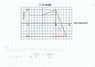# Calculating displacement from velocity-time graph

• Letsgo99

## Homework Statement

Basically they want me to find the displacement for the entire time interval on this graph.
The graph is in the attachment.

## Homework Equations

Displacement is equal to the area under the graph. In this case d=LxW+(BxH)/2+(BxH)/2

## The Attempt at a Solution

My attempted solution is included in the graph. I understand how to do these, my only problem is that I'm not sure what to do with the negative velocity. All the examples in my textbook only deal with positive velocity on problems like this one. I have the area of figure 3 but I'm not sure if I have to include the entire area under the line in figure 1 or just the positive part. With figure two I'm also not sure what to do.

#### Attachments

•scan0001.jpg
42.5 KB · Views: 462